﻿ Symmetric Difference Calculator | Calculate Difference of Two Sets

# Symmetric Difference Calculator for Two Sets

In the set operations, let's calculate symmetric difference. The symmetric difference of set A and set B, is the set containing those elements in exactly one of A and B.

### Symmetric Difference

Like any other operation, a symmetric difference also happens between two sets. Suppose we have two sets, A and B. Their symmetric difference would consist of all the elements present in either A or B but not in the intersection of A and B.

### Representation of Symmetric Difference

When representing the symmetric difference between any two sets, let us assume set A and B are denoted by the symbol ∆.

Mathematically it is represented using: A ∆ B = (A U B) – (A ∩ B)

This representation has been repeatedly explained above. It implies that A ∆ B represents a set that contains the elements from the union of two sets, A and B, minus the intersection between them. Symmetric Difference, in other words, is also called disjunctive union. The symbol ∆ is also a binary operator. Like other binary operators, it takes two operands, two different or identical sets as we know for other operations and their applications for calculating probability between events. The symmetric difference is not only restricted to set operations. We can also use it to calculate the probability of certain multiple events. We can convert the equation written above to an equation with probability.

### Set Operations Table

Set OperationVenn DiagramInterpretation
Union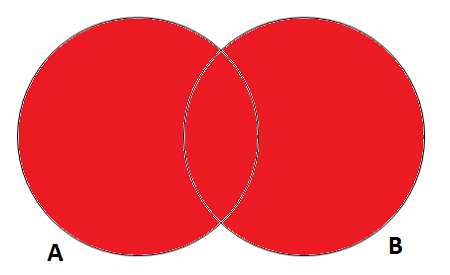A U B, is the set of all values that are a member of A, or B, or both.
Intersection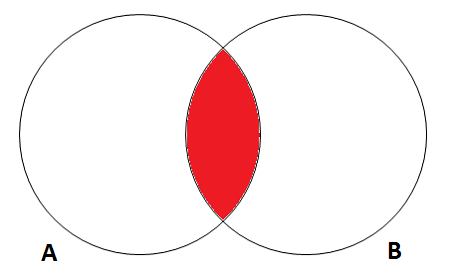A ∩ B, is the set of all values that are a member of both A and B.
Difference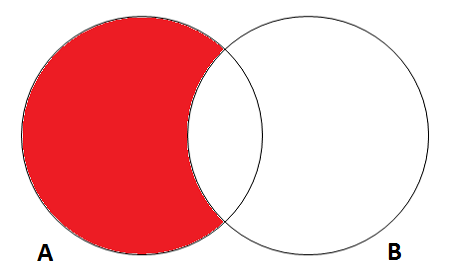A \ B, is the set of all values of A that are not members of B.
Symmetric Difference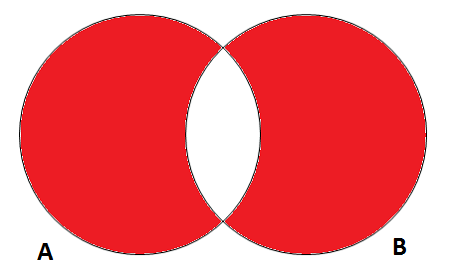A ∆ B, is the set of all values which are in one of the sets but not both.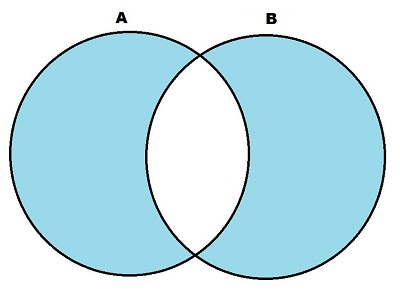### Spreading Knowledge Across the World

USA - United States of America  Canada  United Kingdom  Australia  New Zealand  South America  Brazil  Portugal  Netherland  South Africa  Ethiopia  Zambia  Singapore  Malaysia  India  China  UAE - Saudi Arabia  Qatar  Oman  Kuwait  Bahrain  Dubai  Israil  England  Scotland  Norway  Ireland  Denmark  France  Spain  Poland  and  many more....